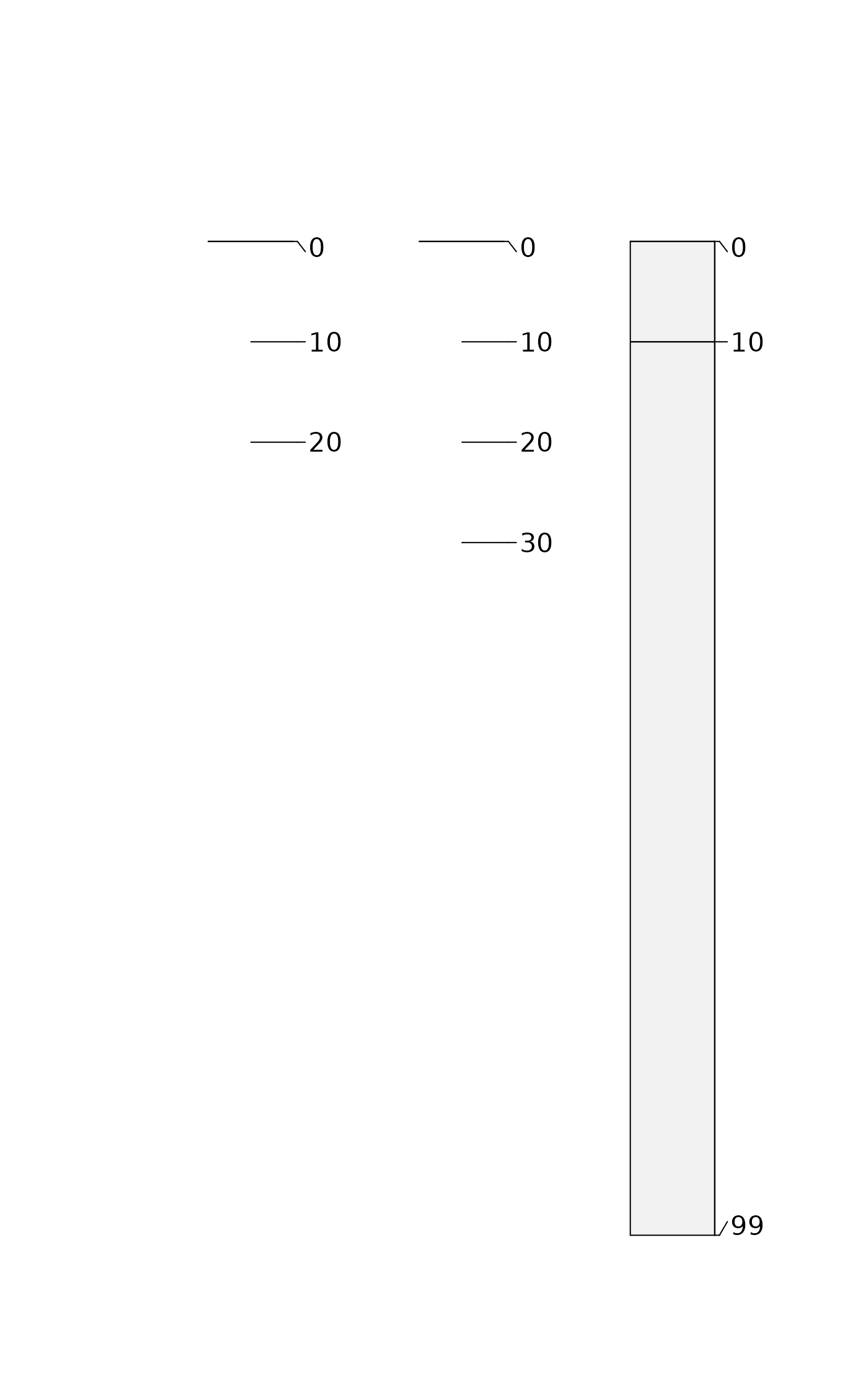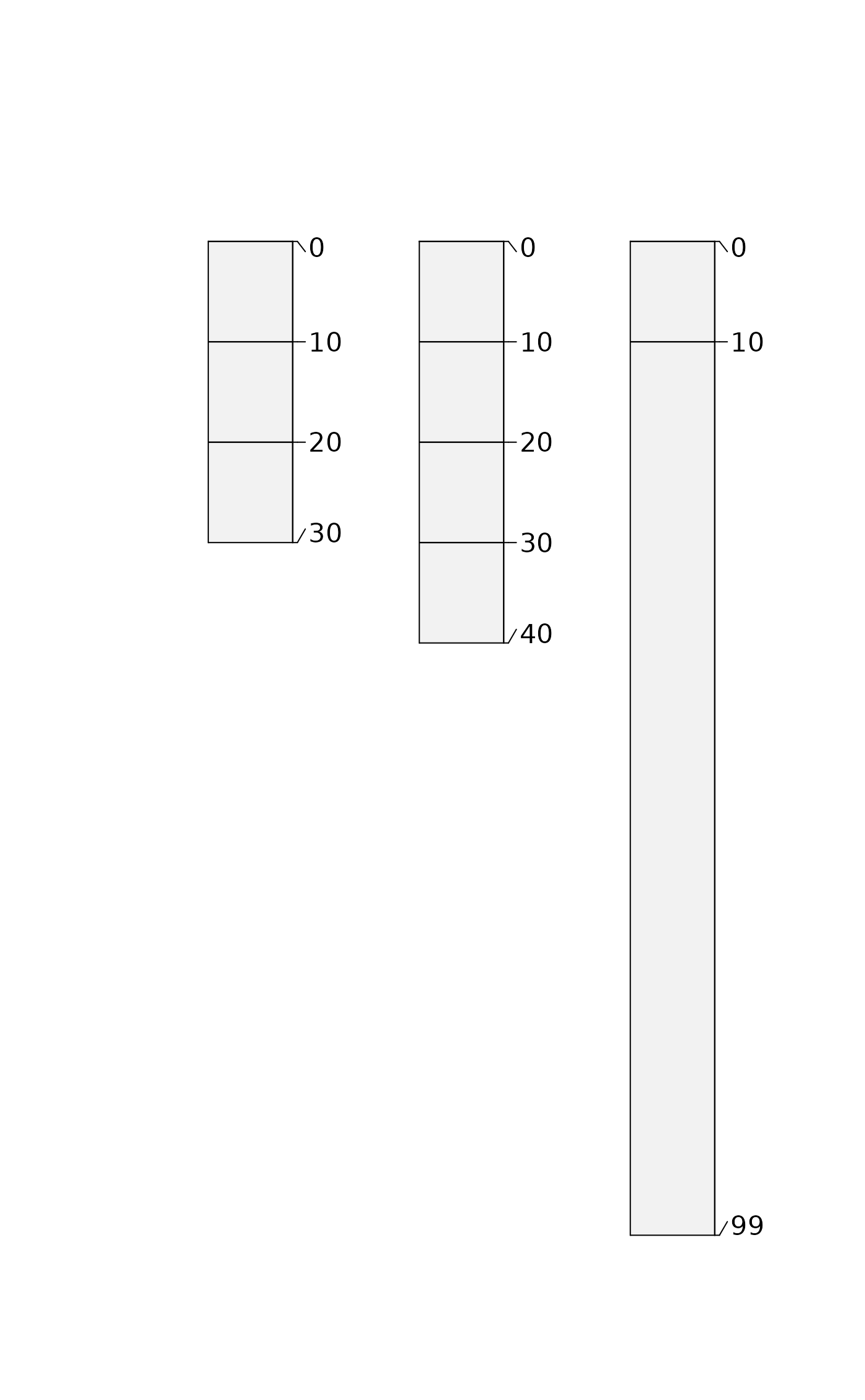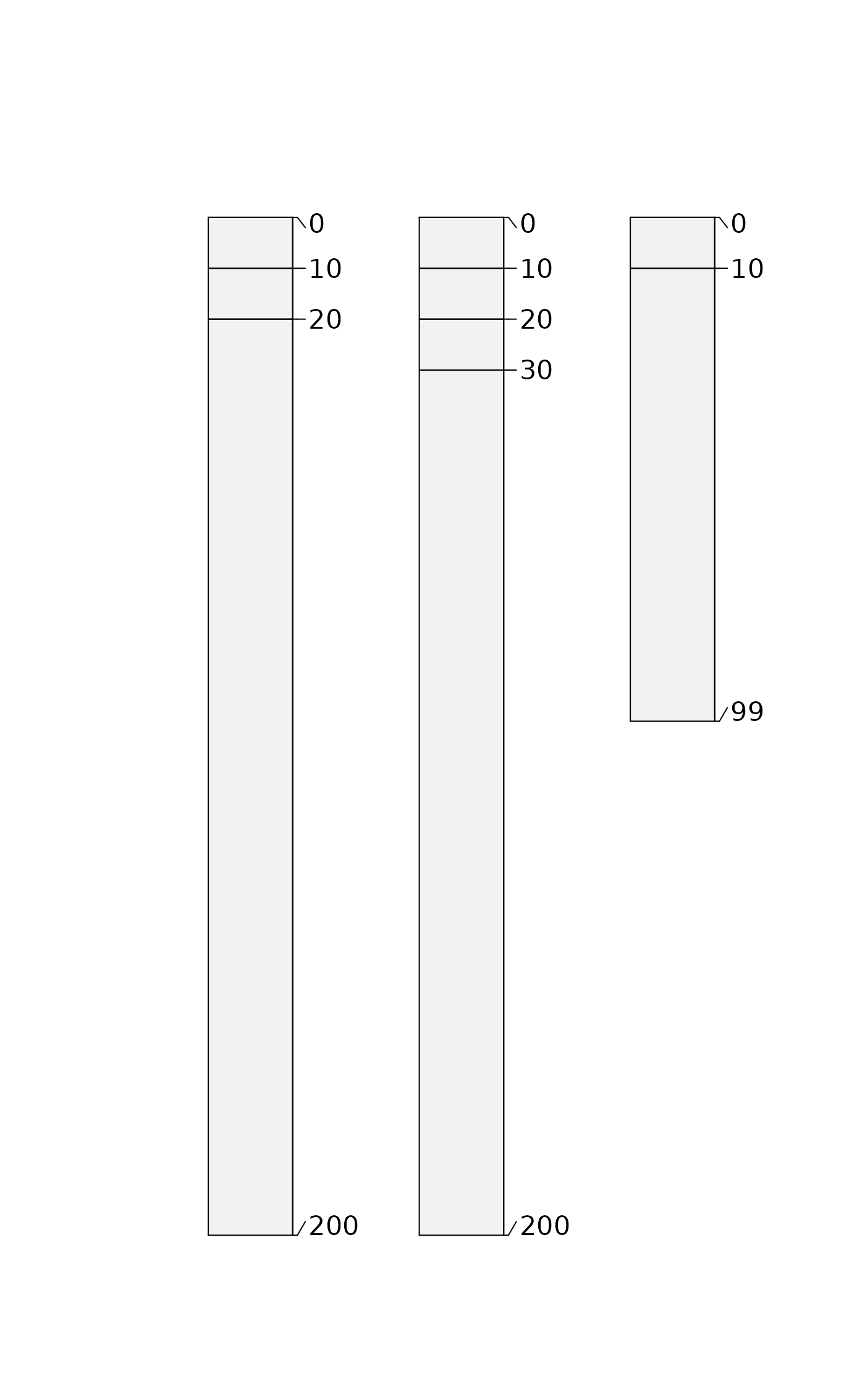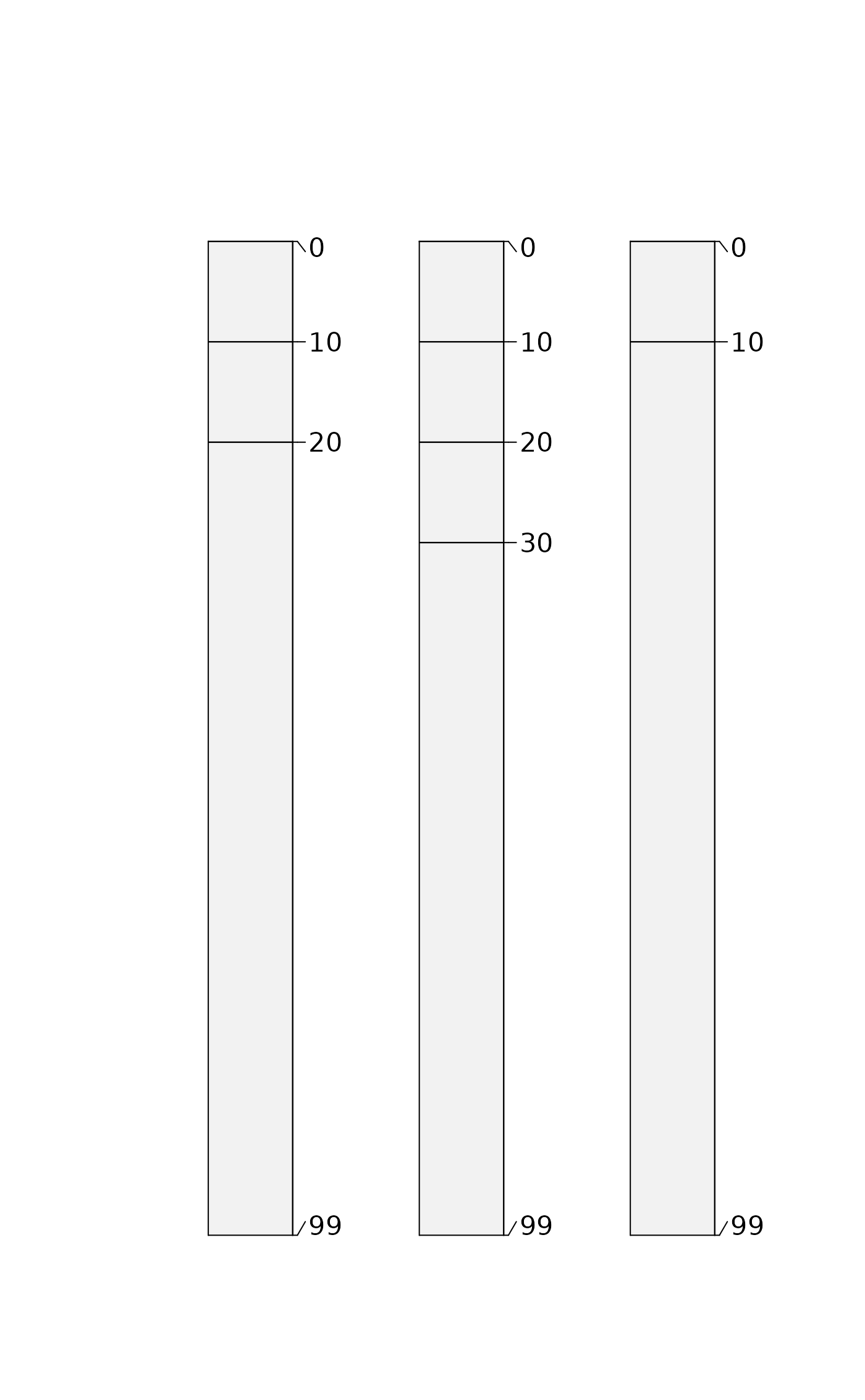Attempt a simple repair of horizon bottom depths in the presence of NA, or in cases where the horizon shares a common top and bottom depth. Both situations are common in pedon description where "contact" (Cd, Cr, R, etc.) was described without a lower depth.

repairMissingHzDepths(x, adj = 10, max.depth = 200)

## Arguments

x

SoilProfileCollection

vertical offset applied to "repair" missing bottom depths when top and bottom depth are equal or bottom depth is missing. (NA to use max.depth)

max.depth

If adj is NA, or the resulting offset sum exceeds max.depth, max.depth is used.

## Value

SoilProfileCollection with a new (logical) horizon-level attribute .repaired marking affected horizons

## Details

This repair is applied to the deepest horizon within a profile as identified by getLastHorizonID, as well as to bottom depths of any horizon that has a horizon below it. Horizon bottom depths are adjusted by adding adj (if non-NA). If the resulting value exceeds max.depth, the max.depth value is returned (if not NA).

## Examples

h <- data.frame(
id = c(1, 1, 1, 2, 2, 2, 2, 3, 3),
top = c(0:2, 0:3, 0:1) * 10,
bottom = c(rep(NA_integer_, 7), c(10, 99))
)

# NA depths result in warnings
suppressWarnings({
depths(h) <- id ~ top + bottom
})

# inspect data before repairs
plot(h)g <- repairMissingHzDepths(h)

# all depth logic now valid
all(checkHzDepthLogic(g)$valid) #>  TRUE # inspect plot(g)# no adj, max.depth only f <- repairMissingHzDepths(h, adj = NA, max.depth = 200) all(checkHzDepthLogic(f)$valid)
#>  TRUE
plot(f)# max.depth defaults to max(x) if too small
f$bottom[c(3,7)] <- NA d <- repairMissingHzDepths(f, adj = NA, max.depth = 20) #> Using max.depth = 99 all(checkHzDepthLogic(d)$valid)
#>  TRUE
plot(d)